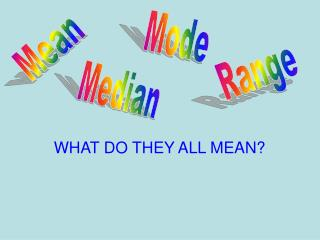DownloadDownload PresentationMean

# Mean

Télécharger la présentation## Mean

- - - - - - - - - - - - - - - - - - - - - - - - - - - E N D - - - - - - - - - - - - - - - - - - - - - - - - - - -
##### Presentation Transcript

1. Mode Mean Range Median WHAT DO THEY ALL MEAN?

2. All of the previous words can be summed up with the phrase MEASURES OF CENTRAL TENDENCY

3. Mean This is what we usually mean when we say “What’s my average.” We could say, “What grade do I normally get?”

4. How is the mean of a set of numbers found? • Add all the numbers in the set together. • Divide the sum by the number of data items in the set. • The quotient is the mean of the set of numbers. • Example: 10, 18, 12 and 16 • Add 10 + 18 + 12 + 16 = 56 • Divide 56 by 4 • The mean is 14

5. Median • Is the number that is in the middle of a set of numbers. • Half the numbers are below the median and half the numbers are above the median

6. How is the median of a set of numbers found? • Arrange the numbers in the set in order from least to greatest. • Count in from the ends. • The middle number is the median of a set of numbers. • If more than one number is in the middle of the set of numbers, the mean of those two numbers is the median of the set of numbers.

7. Your grades on five tests: 95, 87, 92, 100, and 85 Put them in order from least to greatest 85, 87, 92, 95, 100 Your median test grade is 92 – half the time you score below 92 and half the time below 92 The middle number is the median

8. Your grades on six tests: 95, 87, 92, 100, and 94 Put them in order from least to greatest 85, 87, 92, 94, 95, 100 The median is the mean of 92 and 94 Or 92 + 94 = 186 186 divided by 2 = 93 The middle is between 2 numbers

9. How do I identify the mode of a set of numbers? • Arrange the set of numbers in order from least to greatest. • The number that occurs the most often is the mode of that set of numbers.

10. Mode The number in a data set that occurs most often Your grades: 95, 87, 85, 100, 100 Your mode is 100 Most of the time you get a 100

11. You don’t have to have a mode……. Some data sets have no mode or no number that occurs more than others Some might have two modes

12. RANGE Asks the Question: How spread out is my data? Another way to describe data

13. How is the range of a set of numbers identified? • Arrange the numbers in the set in order from least to greatest. • Subtract the lowest number from the highest number in the set of numbers. • The difference of the two numbers is the range of a set of numbers.

14. Back to your test Scores: 95, 87, 92, 100, and 94 Your highest score is a 100 Your lowest score is 87 Your range is 100 – 87 = 13 The range = 13

15. It’s Time To Practice! Number of pets owned by 7 students: 2, 1, 1, 4, 3, 2, 1 2 What is the mean What is the median 2 1 What is the Mode 3 What is the Range

16. 4 ways to describe data The Mean – what we usually think of as the average….. The Median – The middle number in a data set…..50% of the numbers are above and 50% are below the median The Mode – The number that occurs most often….. The Range – the difference between the smallest and bigest number…..